# Duality principle

(diff) ← Older revision | Latest revision (diff) | Newer revision → (diff)
The duality principle in mathematical logic is a theorem on the acceptability of mutual substitution (in a certain sense) of logical operations in the formulas of formal logical and logical-objective languages. Letbe a formula in the language of propositional or predicate logic not containing the implication symbol; a formula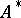is said to be dual to a formulaif it may be obtained fromby replacing ineach occurrence (cf. Imbedded word) of the symbols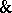,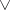,,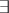by their dual operations, i.e. by the symbols,,, and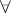, respectively. The duality principle states that if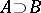is true, thenis true as well. In particular, if two formulasandare equivalent, their dual formulasandare equivalent too. The duality principle is valid for classical systems, and the equivalence and the truth of the formulas involved in its formulation may be understood both in terms of interpretations and in the sense of being deducible in the corresponding classical calculus. The duality principle is no longer valid if the formulas are understood in their constructive sense. For instance, in the language of propositional logic the implication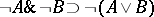is constructively true, and is even deducible in a Heyting formal system, but the converse implication of the dual formula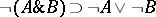is constructively untrue (it is not Kleene-realizable).
The following theorem is closely connected with the duality principle: If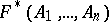is a formula dual to a propositional or predicate formulaconstructed without making use of implications from the elementary propositions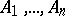, then the formula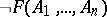is equivalent to the formula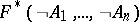in the classical propositional or predicate calculus, respectively.